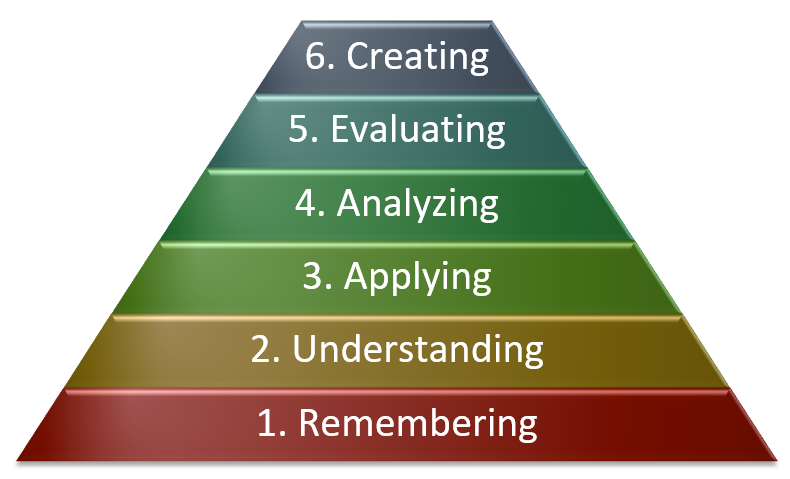# Blooms Taxonomy

In devising assessments, best practice is to have specific learning outcomes in mind as you prepare the assessment, and to align the assessment elements (e.g. questions on an exam, required parts of a report, etc.) directly to those outcomes.  The most widely used system of learning levels (a.k.a. Bloom’s Taxonomy) is provided below, along with examples of associated verbs and exam questions.  See also the page on Constructive Alignment.

This is often presented as a pyramid, with the higher learning levels built on the foundation of lower levels.  (An inverted pyramid can create a stronger visual emphasis on high-level elements and acknowledge that analyzing, evaluating, and creating are somewhat independent.)Starting from the bottom and working up:

### 1. Remembering

• Recall previously learned information
• Verbs: Recall, identify, list, define, locate, name, define,…
• Example: What does the equation x2 + y2 = r 2 describe?
• Exam question types to consider: multiple choice, matching, and fill-in-the-blank

### 2. Understanding

• Comprehending what facts from the “remembering” level mean
• Verbs: Describe, explain, clarify, paraphrase, summarize, discuss…
• Example: Explain why the Pythagoras’s theorem and and a circle share the same equation.
• Exam question types to consider: multiple choice, matching, fill-in-the-blank, short answer

### 3. Apply

• Apply facts, rules, and concepts to do something useful.
• Verbs: Calculate, solve, sketch, determine, use, implement, diagram,…
• Example: Compute the y-coordinates corresponding to x = 0.5 for a circle centred at O with radius 1
• Exam question types to consider: calculations, short answer, sketches

### 4. Analyze

• Break down information into component parts and use that information to do something useful
• Verbs: Classify, compare, determine, diagnose, categorize, organize,…
• Example: Given the following three coordinates, determine the centre and radius of the corresponding circle: (2.29,1.53), (2.73,1.00), (1.41,-1.96)
• Exam question types to consider: calculations, short answer, essay, identify the error

### 5. Evaluating

• Make or defend judgements based on evidence and criteria
• Verbs: Assess, conclude, critique, justify, appraise, defend, test,…
• Example: Judge which shape from several provided is most circular and provide a justification for that choice.
• Exam question types to consider: essay, recommend a choice and defend, identify the error

### 6. Creating

• Combining parts to make something new
• Verbs: Design, formulate, develop, devise, construct, plan…
• Example: Develop the equation for an ellipse centred at O.  (Note: if students had previously seen the equation for an ellipse, this would drop to Level 1, recall.)
• Exam question types to consider: design, generate a plan